# Engineering Samples: The One about Stress Analysis of Riveted Lap Joint

Problem statement: There is a lap joint with one rivet, as shown in the Figure 1 below. The diameter of the rivet is 4 mm. The material of the rivet, as well as the material of pates in joint are given in the table 1 below. Force which is applied to the plate is given in form of distributed force through the cross-sectional area of the plate. Perform the Stress Analysis of the joint in order to verify the durability of the junction.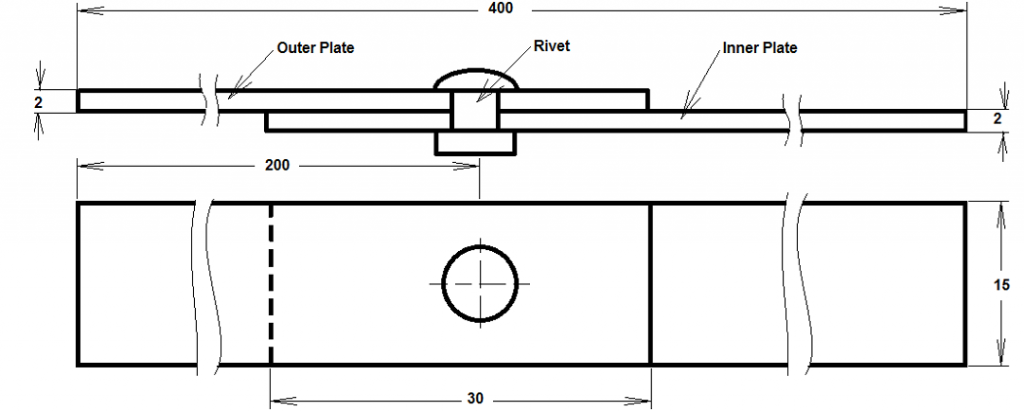Figure 1. Lap joint

Table 1. Material properties

 Material Young’s Modulus Poisson’s Ratio Plate 2024-T3 74 GPa 0.33 Rivet 2117-T4 71.7 GPa 0.33

Solution:

There are four main theories of Failures of Rivet Joints, which are as follows (Dhananjay, n.p.):

• tearing of the plate at an edge;
• tearing of the plate across a row of rivet;
• shearing of rivet;
• crushing of the plate (or) rivet.

The force which was applied in the joint was given in terms of unit force, therefore the total force can be found as multiplication of the unit force on the cross section area of the plate. Since both outer and inner plates have the same dimensions, their cross-section areas are the same too, therefore: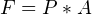Where P is the unit force; A is the cross-sectional area.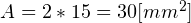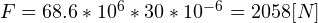The maximum stress due to crushing is: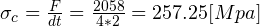Where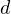is the river diameter;is the plate thickness.
Tearing of the rivet through a row of rivet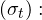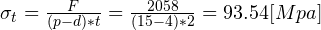Shearing of rivet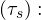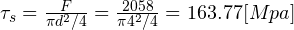Maximum Shear Stress: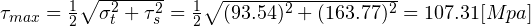As could be seen from the calculations, the maximum stress which occur in the plate due to the loading is 257.25 Mpa, which is less than the yielding stress of the plate material (74Gpa).

The maximum stress which occur in the rivet due to the loading is shearing stress, which is 163,77 Mpa, which is also much lower than the yielding stress of the rivet material, equaled to 71.7 Gpa.

The Efficiency of Riveted joint can be obtained as follows: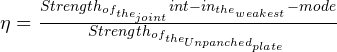Therefore, the efficiency of the joint is: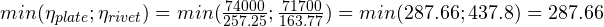Conclusion: The lap join is durable under the given loading, since the maximum stresses which occur in plates and rives are much lower that the maximum allowable stresses.

References:
Dhananjay A Jolhe “Engineering Graphics”, (n.p.). Department of Industrial Engineering. Shri Ramdeobaba Kamla Nehru Engineering College. Nagpur. Extracted from: